# Orthogonal

## Riemannian Electromagnetism

Orthogonal

### The Three Polarisations

In our universe, light in a vacuum is a completely transverse wave: the oscillating electric and magnetic fields that constitute the wave are always orthogonal to the direction in which the wave is travelling through space.In the Riemannian universe, as we discussed in the notes on vector waves, there is also a sense in which the equivalent kind of wave will always be transverse to its direction of propagation: the four-dimensional vector A describing the oscillating field will be orthogonal to the four-dimensional propagation vector k. If the wave is:

A(x) = A0 sin(k · x)

then we must have k · A0 = 0. When that condition is imposed, it allows three independent directions for the wave’s vector. For example, suppose the propagation vector k lies in the xt-plane, as in the diagram on the right. Then if A0 points along the y-axis, along the z-axis, or in the xt-plane itself but at right angles to k, those are three mutually perpendicular directions, all of which are orthogonal to k. (The second possibility isn’t illustrated in the diagram, because we’ve omitted the z direction to allow us to show the t direction.)

But when all these vectors are projected down to three-dimensional space, while the first two directions will remain orthogonal to the direction the wave is travelling, the third ends up parallel (or anti-parallel) to it. In that sense, the Riemannian equivalent of light can include a longitudinal mode, where the field oscillates in the same direction as the wave is travelling. (In the diagram, we show the projections into the xy-plane. Because the vectors we’ve used have no z components anyway, the result is the same as projecting them into three-dimensional space.)

It’s important to stress that these three modes of polarisation — two that appear transverse and one that appears longitudinal — are completely identical when we look at the light itself, as a plane wave in four-space. In every case, k and A0 are orthogonal to each other. It’s only once we choose a particular direction for a time axis and start distinguishing directions in four-space relative to that choice that it means anything to talk about longitudinal polarisation. That said, there is a very real difference here from the situation in our own universe, where there are only two polarisations. In the Riemannian universe, if the equivalent of a polarising filter removed one transverse component of light, two “crossed polarisers” — which in our universe would block light completely — would still transmit the longitudinal component from a randomly-polarised light source.

### Doppler Shift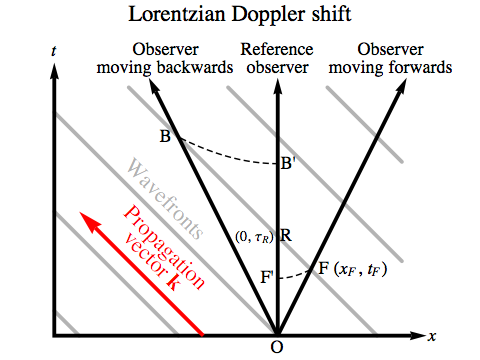The Doppler shift is a well-known effect in which the motion of an observer alters the wavelength and frequency they measure for a light wave.

In the diagram on the right, we show the same sequence of wavefronts passing over three different observers in the Lorentzian universe; the light is coming in from the right. The “reference observer” is assumed to be at rest with respect to the light source, while the other two observers are moving towards and away from the source.

The reference observer will measure a period of OR between successive wavefronts. The observer moving towards the light will encounter wavefronts at the events O and F, but the Euclidean length of OF that we see in the diagram isn’t the time that passes for the observer, which needs to be calculated with the Lorentzian version of Pythagoras’s Theorem. For ease of comparison, we’ve used a hyperbola — a curve of constant proper time in the Lorentzian universe, just as a circular arc is a curve of constant distance in Euclidean space — to bring the interval onto the reference observer’s world line. There we can see unambiguously that OF' is less than OR, so the observer moving forwards will measure the light’s period to be shorter and its frequency to be increased. This is known as blue shift, because blue light is at the high-frequency end of the visible spectrum.

Similarly, the observer moving backwards will encounter wavefronts at the events O and B, and for comparison we’ve transferred the interval to OB', where it’s clear that it’s longer than OR. So the observer moving backwards will measure the light’s period to be longer and its frequency to be decreased. This is known as red shift.

Suppose F has coordinates (xF, tF). If the observer’s velocity is v, then xF = v tF. We’ll write τR for the period of the light, OR, according to the reference observer. Since the wavefront moves from F to R at a speed of 1 in our units, we have:

τR = tF + xF = tF (1 + v)
tF = τR / (1 + v)

The period of the light according to the forward-moving observer is then found from the Lorentzian version of Pythagoras’s Theorem:

τF = √[tF2xF2]
= tF √[1 – v2]
= τR √[1 – v2] / (1 + v)
= τR √[(1 – v) / (1 + v)]

The ratio of frequencies for the Lorentzian blue shift is just the inverse of the ratio of the periods:

νF / νR = τR / τF = √[(1 + v) / (1 – v)]

The Lorentzian blue shift factor doesn’t depend on the particular frequency of the light. Suppose you have a velocity of v = 0.6, sixty percent of the speed of light. Then the blue shift factor is two, and all light coming towards you from straight ahead will have its frequency doubled, compared to when you were at rest. Similarly, all light you see from directly behind will have its frequency halved.

The Riemannian case is a bit more complicated, but let’s start with the same scenario, where one observer is approaching the light source and another is moving away from it — both at less than the speed of the light itself.In this case, we can see clearly from the diagram that OF', the period of the light measured by the observer moving forwards, is greater than OR, while OB', the period measured by the observer moving backwards, is less than OR.

Does this mean the observer moving towards the light sees a “red shift”? At this point we need to stop and ask exactly what kind of language we’re going to use to describe the visible spectrum in the Riemannian universe. This isn’t a matter of philosophical qualms about whether aliens in another universe can experience the same ineffable sense of “redness” and “blueness” as we experience; we can translate their sense of colour into our own vocabulary any way we like, so long as we’re consistent. But whatever the perception of light might feel like for inhabitants of the Riemannian universe, we can’t actually make a perfect translation that respects all the objective, physical properties of light. In our universe, red light has a longer wavelength and a longer period than blue light. In the Riemannian universe, no kind of light has both a longer wavelength and a longer period than any other kind. The relationship is always the opposite: a longer wavelength always means a shorter period.

So we have to make a choice. In the novel Orthogonal the arbitrary choice made was to translate the shortest wavelength of visible light as “violet”, so we will take the term “blue shift” to mean “towards shorter wavelengths”.

With that terminology decided, let’s charge ahead and quantify the easiest thing here, which is still a ratio of periods or frequencies rather than wavelengths. If the speed of the light involved, according to the reference observer, is c, then the wavefronts will be moving in the opposite direction to the propagation vector at a speed of 1/c, because the slope of a line perpendicular to one with slope c is –1/c. The Riemannian version of our previous calculation becomes:

τR = tFxF / (1 / c) = tF (1 – vc)
tF = τR / (1 – vc)
τF = √[tF2 + xF2]
= tF √[1 + v2]
= τR √[1 + v2] / (1 – vc)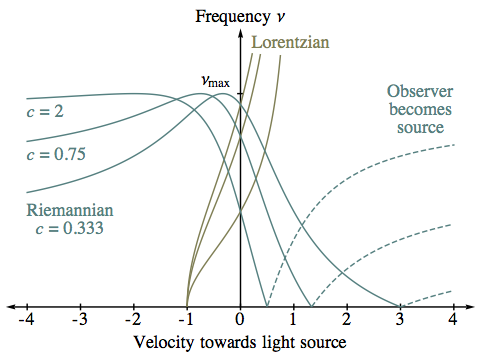We then have a Riemannian “blue shift” that involves a reduction in the observed frequency for the observer moving towards the light source:

νF / νR = τR / τF = (1 – vc) / √[1 + v2]

If the speed of the observer, v, reaches 1/c, then the frequency of light that they measure drops to zero. We see an example of this with the observer in the diagram whose world line is OG. This observer sees the light as having infinite velocity, and the minimum possible wavelength.

What happens if the observer travels forwards at a greater speed than 1/c (for example, the observer whose world line is OH)? The propagation vector coming from the source would appear to them to be pointing back in time, so in any interaction with the light they would consider themselves to be the source, with the direction of the propagation vector reversed.

We can obtain the frequency ratio for the observer moving backwards, away from the light source, simply by putting a negative value for v in the same formula. From the diagram, we can see that something special happens when v = –c: the observer whose world line is OC is keeping pace with the light, giving it a relative velocity of zero. For such an observer, the frequency will reach a maximum, which will be νmax, the maximum frequency possible, as the observer is ploughing directly through the wavefronts.

What happens when the observer starts out-pacing the light, moving backwards even faster than the light is travelling? Our formula for the frequency ratio gives a positive value, which is now less than the maximum, so the light should still be visible. But how can you receive light from a source, when you’re fleeing from the source faster than the light itself? Our assumption is that the light is being constantly produced over an extended period (as would be true for, say, starlight), so it’s not a race between the observer and any single pulse of light. If the observer is moving faster than the light in question, such as the observer whose world line is OD, they’ll start catching up with “old” light that has had enough of a head start to be there waiting for them. And they’ll see this light as arriving from the direction in which they’re travelling, rather than the direction of the light source itself.

As well as the Doppler shift changing the frequency of light, the geometry of the situation means that an observer in motion will measure different angles between light rays than the reference observer, an effect known as aberration. In the Riemannian case, we can understand what’s happening with both Doppler shift and aberration with ordinary Euclidean geometry.

Suppose the reference observer sees light of a particular colour from a multitude of sources spread out evenly across the sky. All the incoming light rays form a cone in four-space, whose axis is the reference observer’s world line. If we project all the propagation vectors onto the reference observer’s idea of space, they will fan out symmetrically in a circle (shown in grey in the diagram below).

An observer at the same location who is moving at some speed v to the right will see light arriving in directions corresponding to the projection of the same rays on the cone onto their own idea of space — which will be tilted with respect to the reference observer’s space. This will have two effects: it will change the lengths of the projections of the propagation vectors, and it will change the angles between those projections. The length of the projection of a propagation vector onto an observer’s idea of space is proportional to the light’s spatial frequency (as we discussed when first considering waves), so a longer projection will mean a shorter wavelength.The diagram shows the result for various velocities for the observer, assuming all the incoming light has a velocity of 0.75 in the reference observer’s frame. The colours here match the translation scheme used in the novel for the visible hues, with dashed lines for ultraviolet and infrared.

In the first example, with v = 0.25, light arriving from the direction in which the observer is travelling is blue-shifted and the sources appear squeezed together in the sky. Light coming from the opposite direction is red-shifted, and spread out across the sky. Phrased this way with red- and blue-shift referring to wavelengths rather than frequencies, this account sounds just like the Lorentzian version.

Once v=c, all light appears to be coming from ahead. At v=1/c=4/3 (not shown), rays from directly ahead will be arriving backwards in time, making them invisible; at v=2, a noticeable portion of the cone has been omitted for this reason.

For v=2, we see something quite odd that would certainly be impossible in Lorentzian physics: light from the same apparent direction, with two different colours, that is actually coming from two different sources. Where rays of red, yellow and green mingle with ultraviolet, the redder light is actually coming from behind (according to the reference observer), but the tilt of the moving observer makes it appear to arrive from ahead, and it is superimposed on light that really is coming from ahead.

At v=∞, all light that the reference observer would say is arriving from ahead is now arriving from the moving observer’s future, making it invisible. The light that is visible here is all from behind, and is crowded together into a region of the sky whose angular width is just that of the cone of incoming rays.

Keep in mind that everything we’ve described here refers to light with just one colour according to the reference observer. Even to an observer who is stationary compared to the average motion of the stars, in the Riemannian universe most stars will appear as colour trails, because the star will have moved slightly between the time when it emitted the red light that is currently reaching the observer and the later time when it emitted the faster, violet light that’s also just arriving. The way Doppler shift and aberration modify these star trails is discussed in the first volume of the novel. You can see illustrations of these effects in the book’s trailers, and there’s also a video tutorial on the subject.

### The Riemannian Electromagnetic Field

So far we’ve been talking about an “oscillating field” A without saying precisely what it is. It turns out that a whole theory of Riemannian electromagnetism can be developed, in a manner that is analogous to the Lorentzian electromagnetism of our own universe. This includes vector fields that we can sensibly call electric and magnetic fields, and a property of matter analogous to electric charge, which results in the matter in question experiencing electromagnetic forces and having the capacity to generate electromagnetic waves.

In this scheme, the field we’ve been calling A is what’s known as the four-vector potential. Just as the electric potential is a quantity whose rates of change across space give us the electric field, the four-vector potential is a vector whose derivatives give us the complete electromagnetic field. Specifically, the electromagnetic field F has components:

Fij = ∂i Aj – ∂j Ai

where i and j range over x, y, z and t. In other words, F is described by a 4×4 matrix, derived from the four-vector potential A.

What is the meaning of that matrix? Suppose we have a particle with charge q, moving along a world line whose tangent in four-space is a unit vector u, the particle’s four-velocity. If we multiply u by the charge q and the matrix F, that tells us the force f that the charged particle experiences:

f = q F u

Of course in Newtonian mechanics force is a three-dimensional vector, but in relativistic physics, whether Riemannian or Lorentzian, it makes more sense to have force as a four-vector. And just as in Newton’s famous equation:

f = m a

(force equals mass times acceleration) the four-vector for force, f, is related to a four-vector for acceleration, a ... which in turn is related to the four-velocity, by:

a = ∂τu

The four-acceleration is the rate at which the four-velocity is changing with respect to proper time, τ which is time measured along the particle’s world line. So if we put all these pieces together, we end up with the following description of the effect of an electromagnetic field F on the motion of a particle with charge q and mass m:

τu = (q / m) F u

The four-velocity u always has a length of 1. Any change in u that was parallel to u itself would alter its length, so the vector describing its rate of change must always be orthogonal to u. This means that F must belong to a special class of matrices, such that multiplying any vector by F gives a result orthogonal to the original vector. It’s not hard to see that this is true for any antisymmetric matrix — that is, any matrix that is equal to the opposite of its own transpose, or Fij = –Fji. Then, using the Einstein summation convention, we have:

u · (F u) = ui Fij uj = ui (–Fji) uj = –u · (F u)

showing that the dot product must be zero. The definition of F in terms of A guarantees that it will be antisymmetric.

What kind of world line will a charged particle follow, if it is moving through a constant electromagnetic field? The easiest case to consider is when the matrix F takes a particularly simple form:

Fij = bi cjbj ci

where b and c are any two non-parallel vectors. (If b is a multiple of c, the right-hand side here becomes zero.) We abbreviate this by writing:

F = bc

The symbol “∧” is called a “wedge product”. In this case, we can rewrite the matrix product of F with a vector u in terms of b and c:

F u = (c · u) b – (b · u) c

Clearly this will always be a vector lying in the plane spanned by b and c. It will also depend solely on the part of u that lies in that plane; if u is entirely orthogonal to the plane (that is, if b · u = c · u = 0), then F u will be zero.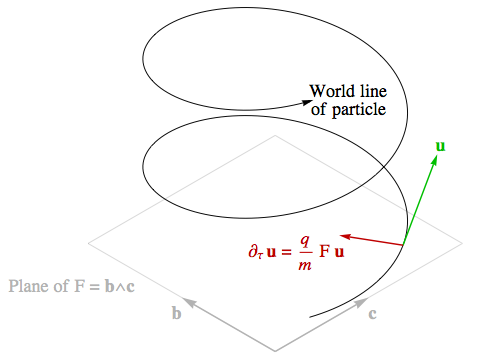The result, in general, will look something like the diagram on the right. With u lying neither entirely in the plane spanned by b and c nor entirely orthogonal to it, the particle will continue to move orthogonally to the plane at a constant rate, while its motion in the directions parallel to the plane will be bent around into a circle. Overall, then, the particle’s world line will be a helix.

If the plane is spanned by two directions that you consider to be directions in space (in other words, the plane is orthogonal to your world line), then the circular part of the motion will look like motion in space to you: either the particle will be circling in a fixed plane, or it will be moving in a helix through space. This is precisely the behaviour that we associate in our universe with a magnetic field. Indeed, in a somewhat old-fashioned kind of particle accelerator known as a cyclotron, a constant magnetic field is used to cause particles to circle this way. The conventions for the description of a magnetic field are that we ascribe to it a direction in space orthogonal to the plane in which the particle is circling.

If the plane includes a direction you consider to be time, then the curvature of the world line will describe the particle accelerating in the plane’s other, spatial, direction. When a charged particle is accelerated this way in our universe, we consider that to be the effect of an electric field. In Lorentzian physics the world line of an accelerating particle is hyperbolic rather than circular, but in all other respects the effect is the same. We ascribe to the electric field a direction in space that lies in the plane.

So an electromagnetic field of the simple form F = bc is seen as:

• a magnetic field perpendicular to both b and c, if b and c are directions in space;
• an electric field with a direction in space that lies in the plane spanned by b and c, if that plane includes your time direction.

If the plane doesn’t fall neatly into either of these categories, F will be seen as containing both an electric and a magnetic field.

Let’s use these descriptions to see what’s happening in our transverse and longitudinal modes of Riemannian light. In general, if:

A(x) = A0 sin(k · x)

then the electromagnetic field is described by a matrix F:

F(x) = (kA0) cos(k · x)

Suppose we fix the light’s propagation vector to be in the xt plane with its speed equal to 1 in the x direction, e.g. k = ex + et. (Actually, it would have to be a multiple of this that met the condition |k| = ωm, but the value of ωm isn’t important in what follows.)

First, let’s consider a wave we’d see as transverse, with A0 = ey. The plane spanned by k and A0 is neither wholly spatial nor does it contain our time axis, et, so the wave will include both electric and magnetic fields. But we can split up F into two parts that, in our coordinate system, correspond to magnetic and electric fields:

F(x) = ((ex + et) ∧ ey) cos(k · x) = (exey) cos(k · x) + (etey) cos(k · x)

The first part, with a plane spanned by ex and ey, is an oscillating magnetic field that points in the z direction. The second part, with a plane spanned by et and ey, is an oscillating electric field that points in the y direction. This is very similar to light in our own universe: the electric field, the magnetic field, and the direction in which the light is travelling are all at right angles to each other.

Next, let’s consider a wave that we’d see as longitudinal, with A0 = exet.

F(x) = ((ex + et) ∧ (exet)) cos(k · x) = 2 etex cos(k · x)

In simplifying the wedge products, we’ve made use of the fact that any vector’s wedge product with itself is zero, and that bc = –cb. The result is an oscillating, purely electric field that points in the x direction, parallel to the motion of the light itself.

### The Corrugated Coulomb Force

The Riemannian Vector Wave equation governs the four-vector potential A for an electromagnetic field in a vacuum — that is, in the absence of any charged matter that might actually give rise to the field in the first place. But we can easily extend the RVW equation to include a “source” term describing the presence of charge, as follows.

 ∂x2A + ∂y2A + ∂z2A + ∂t2A + ωm2 A + j = 0 (RVWS)

The four-dimensional vector j that we’ve added to the equation here has a very simple meaning. Suppose there is some charged material present in the region of interest, and an observer at rest with respect to that material measures the density of charge to be ρ. If the four-velocity of the material is u, we define:

j = ρ u

Now, u is a unit vector tangent to an object’s world line. But there are two such vectors at every point on any world line, pointing in opposite directions. How do we choose the right one? In one direction the charged material will look positively charged to an observer who takes that choice of u as their time axis; in the other direction the same material will look negatively charged. Swapping our choice will multiply both ρ and u by minus one, cancelling out the change. So it makes no difference which choice we make.

We call the vector j the four-current. If an observer who is not at rest with respect to the charged material measures the components of j, they will see both a charge density, jt, and a current density with components jx, jy and jz, describing the motion of charge.

One of the simplest and most important solutions to this equation is known as the Coulomb potential. This is the potential of a particle with a charge q that occupies a single point, at rest in some coordinate system. Of course this is an idealisation, and it has the slightly messy mathematical feature of involving an infinite density of charge along the particle’s world line. Nevertheless, the solution is not too hard to find. The details of how this can be done are in the extra notes on this topic; here we’ll just take it as given. If r is the distance from the particle, the four-vector potential is:

A(r) = [q / (4 π r)] cos(ωm r) et

When A has only a t component like this, and A itself is unchanging in time, it’s simpler to forget about the four-vector potential and just work with the electric potential, φ, which is the opposite of At.

 Coulomb potential φ(r) = –[q / (4 π r)] cos(ωm r) (Riemannian) φ(r) = q / (4 π r) (Lorentzian)

The electric potential is the potential energy of a positive “test particle” with one unit of charge — and as with any potential energy that varies from place to place, it gives rise to a force. The component of the force in any direction is the opposite of the rate of change of φ in that direction (a particle will accelerate in the opposite direction to an increase in potential energy, just as a stone will slide down a hill):

f = –(∂xφ ex + ∂yφ ey + ∂zφ ez)

which we abbreviate using the gradient symbol “∇” as:

f = –∇φ

The simple relationship we’ve claimed between φ and At can be verified by checking that they both give rise to exactly the same force on a test particle.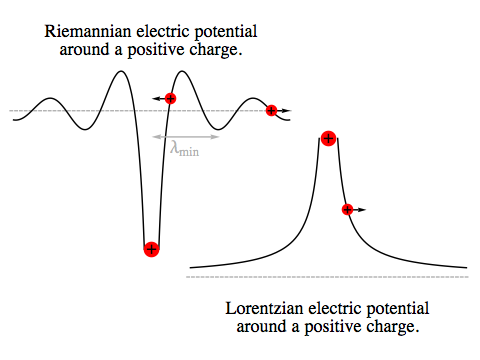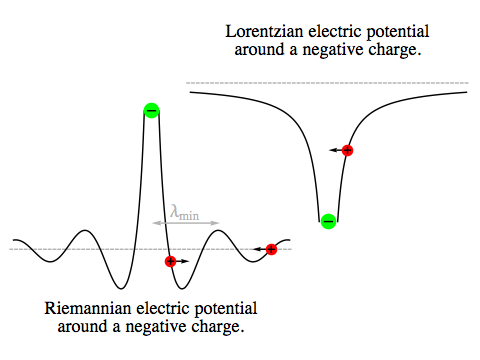What does φ look like? The diagram on the upper right shows the electric potential around a positive charge, in both Riemannian and Lorentzian electromagnetism. The diagram below shows the potential around a negative charge.

There are two striking differences between the Riemannian and Lorentzian cases. In our own universe, it’s widely known that opposite charges attract, while like charges repel. But in the Riemannian universe, the potential near a positive charge is a well, not a mountain, so if two positive charges are sufficiently close they will attract. Opposite charges, if sufficiently close, will repel.

The second difference is that we have to specify the distance between the charges in order to say whether they attract or repel each other. In the Lorentzian universe, the electrostatic force simply tapers off with distance; in the Riemannian universe, the direction of the force also alternates with distance. We can see from the form of our solution for φ that it does this with a spatial frequency equal to the maximum possible frequency of light, νmax (since ωm = 2 π νmax). Equivalently, its undulations have a wavelength equal to the minimum possible wavelength of light, λmin = 1 / νmax.

Now, it’s not hard to verify that our solution for A satisfies the RVWS equation, and the alternating potential really shouldn’t surprise us too much, since we’ve already seen that a plane wave in the ultraviolet limit also involves an electromagnetic field that is unchanging over time while oscillating with a spatial frequency of νmax. The equation requires the sum of the squares of the frequencies in all directions to be a constant, so any static field, unchanging in time, must oscillate in space.

But how can we be confident that the equation itself is correct? If we changed the sign of j in the equation, we would then have the opposite of our original solution, and end up with like charges that repel, close up. What’s to stop us from proposing that the laws of electromagnetism in the Riemannian universe take that form instead?

The simplest answer is to remind ourselves of something we discussed in the notes on energy and momentum, when we realised that in the Riemannian universe relativistic total energy has the opposite sense to potential and kinetic energy. In the Lorentzian universe, an electromagnetic field possesses energy, in proportion to the square of the field’s strength. Given a positive and negative charge, that energy is reduced as the two charges draw closer together, because they will increasingly cancel each other’s fields. But in the Riemannian universe, minimising the potential energy means maximising the field’s energy. For like charges that are sufficiently close, bringing them even closer superimposes their identically shaped fields in an ever-better match, reducing the amount of cancellation between the two particles’ fields and increasing the overall field energy.

### Unpopular Electronics

In our universe, the tiny electric fields that surround charged particles such as electrons can easily accumulate to the point where they have a visible effect. Rub a plastic pen a dozen times against the right kind of clothing, and it will end up with an excess charge that gives rise to an electric field strong enough to lift a scrap of paper against gravity. (The paper itself has no net charge, but the pen’s electric field creates a separation of charge across the surface of the paper.) It doesn’t matter precisely how the excess charge is distributed over the pen’s surface; so long as there is an excess, every part of it will work together to increase the strength of the electric field around the pen — and that electric field will be both persistent in time and consistent across space, pointing in more or less the same direction over an extended region, and remaining unchanged until the charge slowly leaks away.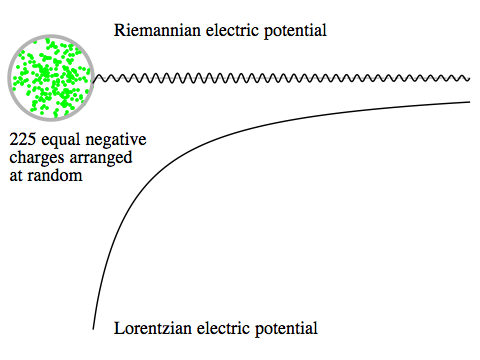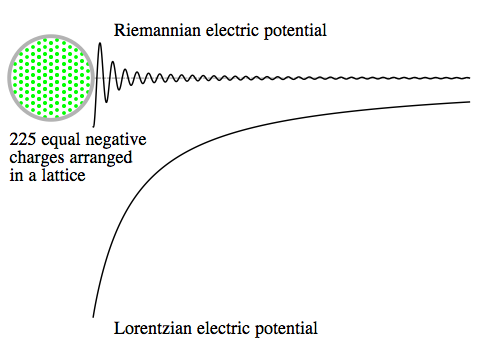In the Riemannian universe, thanks to the oscillation over space of the Coulomb potential the effect of an excess charge is very different. A large number of identical particles arranged haphazardly (as in the diagram on the right) will produce contributions to the potential at a nearby point that are both positive and negative, largely cancelling each other out. Of course the cancellation won’t be perfect, but whatever field remains will be a series of closely spaced hills and valleys, rather than the single, persistent slope that we get in the Lorentzian case.

An orderly array of charges (as in the diagram on the lower right) can result in less cancellation at some points than a random arrangement, but again it would produce an undulating potential landscape. It’s certainly possible for a positive test particle to be repelled by this collection of charges, if it starts from rest at the right location, but the effect would not be as simple to reproduce as the equivalent under Lorentzian physics. What’s more, any object placed in this field that consists of many particles rather than just one will experience a sum of individual forces that depend on the field in a multitude of slightly different locations, leading to further cancellation.

The upshot is that electrostatic phenomena will be sensitive to the detailed, microscopic arrangement of charge, and in general they will be much more difficult to detect and exploit than they are in our universe.

Similar effects will apply to magnetism. In our world merely aligning the direction of a vast number of small magnetic dipoles is enough for their fields to reinforce each other; that’s how a permanent magnet works. But in the Riemannian universe, even when the dipoles themselves are all lined up, as you move across space the field due to each dipole will switch back and forth between two opposing directions, and at any one point there will be cancellation between the contributions from different dipoles at slightly different distances.

Now, although these problems rule out the kind of easy, low-tech experiments that gave us our first hints about the nature of electromagnetism, they are not completely insurmountable. The very small wavelength of the static, unchanging fields we’ve described that makes everything so finicky and prone to cancellation will gradually give way to larger wavelengths as oscillations in time are introduced. Electronic components that would need to be engineered to extremely high precision in order to function in direct current circuits will have far more tolerance for imperfections if they are working with alternating current of a sufficiently high frequency.

What counts as “a sufficiently high frequency”? The field’s wavelength won’t grow to ten times the minimum wavelength until the frequency ω reaches 0.995 ωm, so the trade-off for gaining any useful degree of tolerance for spatial imprecision is the need to deal with, essentially, the highest frequency of electromagnetic radiation that can propagate in the Riemannian universe. That will present its own technical challenges — but they won’t be quite the same as the difficulties of working with high frequencies in our own universe, some of which are actually related to the wavelength.

There is extra material on this topic for readers who don’t mind a slightly higher level of mathematics.Orthogonal / Riemannian Electromagnetism / created Wednesday, 6 April 2011